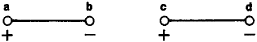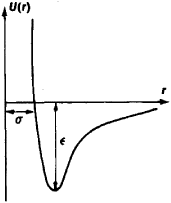# Molecular Interaction

Also found in: Acronyms.
The following article is from The Great Soviet Encyclopedia (1979). It might be outdated or ideologically biased.

## Molecular Interaction

interaction between electrically neutral molecules or atoms; it determines the existence of liquids and molecular crystals and the difference between real and ideal gases and is manifested in various physical phenomena. Molecular interaction depends on the distance r between the molecules and usually is described by the potential energy of interaction U(r), the intermolecular potential, since it is precisely the average interaction potential that determines the state of matter and many of its properties.

Molecular interaction was first taken into consideration by J. D. van der Waals (1873) in explaining the properties of real gases and liquids. He proposed that at small intermolecular distances r there exist forces of repulsion, which with increasing distance are replaced by forces of attraction. On the basis of these concepts, without even considering the quantitative dependence of molecular interaction on distance, he derived the van der Waals equation for the state of a real gas.

Molecular interaction is electrical in nature and consists of forces of attractive forces (orientation, induction, and dispersion forces) and repulsive forces.

Orientation forces act between polar molecules (that is, molecules that have electric dipole moments). The attractive force between two polar molecules is a maximum when their dipole moments lie in the same line (Figure 1). This force arises because the distance between unlike charges is slightly smaller than the distance between like charges. Consequently, the attraction of the dipoles exceeds their repulsion. The interaction of dipoles depends on their relative orientation; therefore, the forces of dipole interaction are called orientation forces. Random thermal motion continually changes the orientation of polar molecules, but calculations show that the average value of the force for all possible orientations has a definite nonzero value. The potential energy of the orientation interaction is Uor(r) ˜ p1p2/r6, where p1 and p2 are the dipole moments of the interacting molecules. The corresponding force of interaction is For ˜ r7. The force For decreases with distance much more quickly than does the coulomb force of interaction of charged particles (Fcoul ˜ r-2).Figure 1. The two electric dipoles ab and cd attract each other in this relative position, since the opposite charges at points b and c interact more strongly than do the like charges at points a and c (and also at b and d)

Induction (or polarization) forces act between polar and non-polar molecules. The polar molecule creates an electric field that polarizes the nonpolar molecule, whose electric charges are symmetrically distributed throughout the volume. Positive charges are displaced in the direction of the electric field, and negative charges are displaced in the opposite direction. As a result, a dipole moment is induced in the nonpolar molecule.

In this case the energy of molecular interaction is proportional to the dipole moment pi of the polar molecule and the polarizability a 2, which characterizes the ease with which the other molecule can be polarized: Uind(r) ˜ P1α2/r6. This energy is called the induction energy, since it appears as a result of the molecular polarization induced by electrostatic induction. Induction forces (Find ˜ r—7) also act between polar molecules.

Dispersion molecular interaction acts between nonpolar molecules. The nature of this interaction was fully elucidated only after the development of quantum mechanics. In atoms and molecules the electrons move around the nuclei in an intricate manner. The time average of the dipole moment of a nonpolar molecule is found to be zero, but at each instant the electrons occupy a certain position. Therefore, the instantaneous value of the dipole moment (for example, that of a hydrogen atom) is nonzero. The instantaneous dipole creates an electric field that polarizes the neighboring molecules. Interaction of the instantaneous dipoles occurs as a result. The energy of interaction of nonpolar molecules is the average result of the interaction of all possible instantaneous dipoles with the dipole moments that they induce in neighboring molecules. The potential energy of the dispersion molecular interaction is Udis(r) ˜ α1α2, and Fdis ˜ r-7 (here α1 and α2 are the polarizabilities of the interacting molecules). Interaction of this type is called dispersion interaction because it is determined by the same molecular properties as is the dispersion of light in matter. Dispersion forces act between all atoms and molecules, since the mechanism by which they appear is independent of whether the molecules (or atoms) have permanent dipole moments. These forces are usually greater in magnitude than orientation or induction forces. The quantity For is greater than Fdis only for molecules with large dipole moments—for example, water molecules, for which the difference is a factor of 3. However, for the interaction of such polar molecules as CO, HI, and HBr the dispersion forces exceed all. other forces by a factor of tens or hundreds. It is significant that all three types of molecular interaction decrease in the same way with distance:

U = Uor + Uind + Udis ˜ r-6

Forces of repulsion act between molecules at very small distances, where the filled electron shells of the atoms making up the molecules come into contact. The Pauli exclusion principle in quantum mechanics prohibits the interpenetration of filled electron shells. The repulsive forces that arise here are more dependent on the molecular types than are the attractive forces. The assumption that the potential energy of the repulsive forces Urep increases with decreasing distance according to the law Urep (r) ˜ r-12, and Frep ˜ r-13, leads to good agreement with experimental data.

If we assume that U(r) = 0 as r — ∞ and that the energy of attraction varies with distance as r-6 and the energy of repulsion as r-12, then the U(r) curve will have the shape depicted in Figure 2. The distance at which the forces of interaction of the molecules are equal to zero corresponds to the minimum of the potential energy curve.Figure 2. Dependence of the Lennard-Jones potential U(r) of molecular interaction on the distance r between the molecules. The distance r = σ is the shortest possible distance between the molecules at rest, and ε is the depth of the potential well (the cohesion energy of the molecular pair).

It is impossible in practice to calculate U(r) with high accuracy on the basis of quantum mechanics for the great diversity of possible pairs of interacting molecules. Neither has it yet been possible to measure experimentally the force of interaction at intermolecular distances. Therefore, a formula for U(r) is usually selected in such a way that the calculations made with it are in good accord with experiment. The formula

U(r) = 4ε[(σ/r)12 - (σ/r)6

called the Lennard-Jones potential, is most often used. The quantities cr and € in the formula are determined experimentally on the basis of the dependence on cr and of the properties of the substance (for example, the diffusion coefficient, thermal conductivity, or viscosity).

### REFERENCES

Radchenko, I. V. Molekuliarnaia fizika. Moscow, 1965.
Coulson, C. “Mezhatomnye sily—ot Maksvella do Shredingera.” Uspekhi fizicheskikh nauk, 1963, vol. 81, no. 3.
Hirschfelder, J., C. Curtiss, and R. Bird. Molekuliarnaia teoriia gazov i zhidkostei. Moscow, 1961. (Translated from English.)

G. IA. MIAKISHEV

The Great Soviet Encyclopedia, 3rd Edition (1970-1979). © 2010 The Gale Group, Inc. All rights reserved.
References in periodicals archive ?
[[Epsilon].sub.ij] = Molecular interaction energy between a segment of molecule i and a segment of molecule j, kJ/kmol.
Increase in the value of relaxation time and ultrasonic absorption with concentration can be explained in terms of the motion of the molecular interaction forces.
Nevertheless, we attempted to calculate the molecular interaction energies keeping in mind that the results are only indicative and that the actual values must be handled with caution.
Furthermore, there is no molecular interaction study performed computationally to establish the conformational changes and reveal the binding domains of A[beta]42 and ectodomain of p75NTR receptor.
Initium has developed and launched molecular interaction analysis systems that are simple and easy-to-use, since it was established.
Experts fromCardiffUniversityand Kings College Londonworked together to isolate a T-cell from a patient with Type 1 diabetes to view a unique molecular interaction, which results in the killing of insulin-producing cells in the pancreas.
Other chapters describe chromatography as more than a method of separation, but as a unified science; profiles of early pioneers and builders, Nobel Prize awardees, and their research; the multiple branches of the field; advances in column technology and its validation; key institutions; using chromatographic separations as molecular interaction amplifiers; and the future of the field and its contributions in systems biology, genomics, and proteomics.
The ratios of the components that define the samples were chosen to ensure sufficient molecular interaction while keeping the soy protein in great excess.
This paper deals with the structure of the molecular interaction as studied by X-ray crystallography and fluorescence anisotropy, of the RXR binding domain with a rexinoid agonist (CD3254, see below, and its analogues).
Simulations observe the behavior of a model system in a given thermodynamic state under atomic and/or molecular interaction rules (electron wave functions and/or force fields), which are given by the programmer to approximate the real interactions.

Site: Follow: Share:
Open / Close